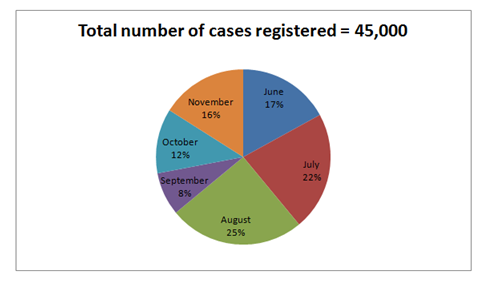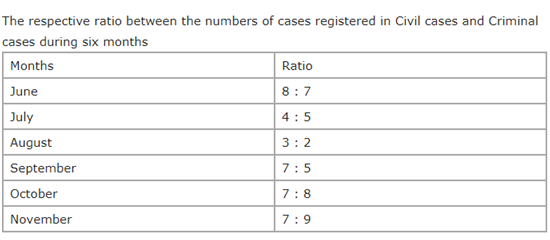# CWC/FCI Prelims 2019 – Quantitative Aptitude Questions (Day-31)

Dear Readers, Exam Race for the Year 2019 has already started, To enrich your preparation here we are providing new series of Practice Questions on Quantitative Aptitude – Section for CWC/FCI Exam. Aspirants, practice these questions on a regular basis to improve your score in aptitude section. Start your effective preparation from the right beginning to get success in upcoming CWC/FCI Exam.

[WpProQuiz 5774]

Directions (1 – 5): What value should come in place of question mark (?) in the following questions?

1) 156 × 684 ÷ (12 % of 650) = 56 × 456 ÷ 3 – ? + 5100

a) 12244

b) 13578

c) 14780

d) 16356

e) None of these

2) (3/5) of 2295 × 75 ÷ 15 – ? = 129 ÷ 3 + 157

a) 7154

b) 7328

c) 6892

d) 6685

e) None of these

3) 5 (7/8) + 3 (½) + 7 (¾) – 1 (1/8) =? + 2 (¼)

a) 10 (1/4)

b) 13 (3/4)

c) 12 (5/6)

d) 11 (½)

e) None of these

4) 16384 × √3136 ÷ 8 – 202 =? – 562

a) 3856

b) 3428

c) 3632

d) 3050

e) None of these

5) (729)2 × (117)2 ÷ (13)2 × 35 = 3?

a) 25

b) 21

c) 24

d) 22

e) None of these

Directions (6-10): Study the following pie-chart and table carefully and answer the questions given below

Percentage wise distribution of the number of case registered by court during six years6) If the court charged a fee of 456 on each case registered in Criminal cases in the month of September, what was the total fee collected on the Criminal cases in the same month?

1. 694900
2. 644800
3. 648400
4. 684000
5. 670500

7) If 35% of the case registered in Civil cases during October were not have proper witness, how many cases registered in Civil cases during that month were have proper witness?

1. 1028
2. 1638
3. 1728
4. 1362
5. 1475

8) The number of case registered in civil cases during June is approximately what percent of the number of case registered in civil cases during November?

1. 120
2. 135
3. 144
4. 125
5. 130

9) What is the respective ratio of the number of case registered in Criminal cases during June to those case registered during November of the same case?

1. 119 : 178
2. 135 : 136
3. 119 : 135
4. 119 : 120
5. 135 : 119

10) What is the total number of case registered in Criminal cases during July and August together?

1. 10,000
2. 20,000
3. 15,500
4. 25,500
5. 12, 000

Direction (1-5) :

156 × 684 ÷ 12 % of 650 = 56 × 456 ÷ 3 – x + 5100

(156*684) ÷ [(12/100)*650] = (56*456)/3 – x + 5100

(156*684)/78 = 8512 – x + 5100

X = 8512 + 5100 – 1368

X = 12244

(3/5) of 2295 × 75 ÷ 15 – x = 129 ÷ 3 + 157

(3/5)*2295*(75/15) – x = (129/3) + 157

6885 – x = 43 + 157

6885 – 43 – 157 = x

X = 6685

5 (7/8) + 3 (½) + 7 (¾) – 1 (1/8) – 2 (¼) = x

X = (5 + 3 + 7 – 1 – 2) (7/8 + ½ + ¾ – 1/8 – ¼)

X = 12 [(7 + 4 + 6 – 1 – 2)/8] = 12 (14/8) = 13 (6/8)

X = 13 (3/4)

√16384 × √3136 ÷ 8 – 202 = x – 562

(128*56)/8 – 400 = x – 3136

896 – 400 + 3136 = x

X = 3632

(729)2 × (117)2 ÷ (13)2 × 35 = 3x

(36)2 × (117*117)/(13*13) × 35 = 3x

312 × 9 × 9 × 35 = 3x

312 × 32 × 32 × 35 = 3x

312 + 2 + 2 + 5 = 3x

3x = 321

X = 21

Direction (6-10) :

Number of case registered in September = 45000 × 8/100 = 3600

Number of case registered in Criminal cases in September = 3600 × 5/12

= 1500

Total fees collected in Criminal cases in September = 1500 × 456 = 684000

Number of case registered in October = 45000 × 12/100 = 5400

Number of case registered in Civil cases in the year of October = 5400 × 7/15 = 2520

Numbers of case registered in October by Civil cases have proper witness

= 2520 × 65/100

= 2520 × 0.65 = 1638

Number of case registered in June = 45000 × 17/100 = 7650

Number of case registered in Civil cases in June = 7650 × 8/15 = 4080

Number of case registered in November

= 45000 × 16/100 = 7200

Number of case registered in Civil cases in November = 7200 × 7/16 = 3150

Required per cent = 4080/3150 × 100 = 129.52

= 130

Total number of case registered in June = 45000 × 17/100 = 7650

Case registered in Criminal cases in June = 7650 × 7/15 = 3570

Total number of case registered in November = 45000 × 16/100 = 7200

Case registered in Criminal cases in November = 7200 × 9/16 = 4050

Required ratio = 3570/4050

= 357/405

= 119/135

=119:135

Number of case registered in July = 22/100 × 45000 = 9900

Number of case registered in August = 25/100 × 45000 = 11250

Number of case registered in Criminal cases in July = 9900 × 5/9 = 5500

Number of case registered in Criminal cases in August = 11250 × 2/5 = 4500

Total number of case registered in July and August by Criminal cases

= 5500 + 4500 = 10000